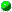`All Packages  Class Hierarchy  This Package  Previous  Next  Index`

# Class jnt.linear_algebra.CoordinateSparseMatrixDouble

```java.lang.Object
|
+----jnt.linear_algebra.CoordinateSparseMatrixDouble
```

public class CoordinateSparseMatrixDouble
extends Object
A coordinate-storage representation of sparse matrices in which the nonzeros at location A[i][j] are stored explicity. No order is imposed on the storage order. and basic algebraic operations.

The nonzero value, together with its offset are stored. Thus, the 5-element matrix

```
0   1   0
4   2   0
0   0   7
```
could be represented by the following contstructor:
```double val[] = new double;
int    row[] = new int;
int    col[] = new int;

val = 1.0;  col = 0; row = 1;
val = 4.0;  col = 1; row = 0;
val = 2.0;  col = 1; row = 1;
val = 7.0;  col = 2; row = 2;

CoordinateSparseMatrixDouble S = new
CoordinateSparseMatrixDouble(3, 3, 4, val, row, col);
```
or, equivalently
```CoordinateSparseMatrixDouble S = new
CoordinateSparseMatrixDouble(3, 3, 4);

for (int i=0; i<3; i++)
S.set_ith_element(i, row[i], col[i], val[i]);
```

General set/get functions which treat the matrix as dense, are available but are not very efficient (i.e. they have an O(nz) cost).

```S.set(i,j, val);        // equivalent to A[i][j] = val;
v = S.get(i,j);         // equivalent to v = A[i][j];
```

The elements are unoreded, and offsets begin at 0. Note, also, that coordinate matrix representations are not unique -- the elements are unordered, and explicit zeros could be stored.

Version:
0.5
Author:
Roldan Pozo

##CoordinateSparseMatrixDouble(int, int)
Initialize a sparse vector of a given dimension.CoordinateSparseMatrixDouble(int, int, int)
Initialize a sparse vector of a given dimension and nonzeros.CoordinateSparseMatrixDouble(int, int, int, int[], int[], double[])
Initialize a sparse vector of dimension N, with nz nonzeros, given values from `val[]` and integer positions in `ind[]`.

##col()
Return vector of column indices as a contiguous array.col(int)copy()
Create a copy of an existing sparse vector.M()
Return the first dimension of the sparse matrix.mult(double[])
Compute A*xmult(double[], double[])
Compute y += A*x;N()
Return the second dimension of the sparse matrix.num_nonzeros()
Return the number of number of nonzeros in sparse matrix.print()readfile(String)
Read a sparse matrix from an ASCII text file.readfile(String, int)
Reads sparse form file, similar to readfile(String) but has an optional base-offset parameter to denote the starting position of matrix indices.row()
Return vector of row indices as a contiguous array.row(int)val()
Return vector of nonzero values.val(int)write()write(CoordinateSparseMatrixDouble)

##CoordinateSparseMatrixDouble
``` public CoordinateSparseMatrixDouble(int M,
int N)
```
Initialize a sparse vector of a given dimension. No storage space for nonzeros is actually created.

Parameters:
N - the dimension of the vector (not the number of nonzeros.)CoordinateSparseMatrixDouble
``` public CoordinateSparseMatrixDouble(int M,
int N,
int nz)
```
Initialize a sparse vector of a given dimension and nonzeros.

Parameters:
N - the dimension of the vector.
nz - the number of nonzeros.CoordinateSparseMatrixDouble
``` public CoordinateSparseMatrixDouble(int M,
int N,
int nz,
int row[],
int col[],
double val[])
```
Initialize a sparse vector of dimension N, with nz nonzeros, given values from `val[]` and integer positions in `ind[]`.

Parameters:
N - the dimension of the vector.
nz - the number of nonzeros.
val[nz] - vector of nonzero values
ind[nz] - vector of positions of elements in val[].

##copy
``` public CoordinateSparseMatrixDouble copy()
```
Create a copy of an existing sparse vector.val
``` public final double[] val()
```
Return vector of nonzero values.

NOTE: this access method is here for efficiency. It should be used by 'trusted' apps only, because it essentially bypasses the 'private' status of these variables.val
``` public final double val(int i)
```row
``` public final int[] row()
```
Return vector of row indices as a contiguous array.

NOTE: this access method is here for efficiency. It should be used by 'trusted' apps only, because it essentially bypasses the 'private' status of these variables.row
``` public final int row(int i)
```col
``` public final int col(int i)
```col
``` public final int[] col()
```
Return vector of column indices as a contiguous array.

NOTE: this access method is here for efficiency. It should be used by 'trusted' apps only, because it essentially bypasses the 'private' status of these variables.mult
``` public final double[] mult(double x[])
```
Compute A*xmult
``` public final void mult(double y[],
double x[])
```
Compute y += A*x;

Parameters:
x - the vector to multiply
y - the vector to updateprint
``` public void print()
```write
``` public static void write(CoordinateSparseMatrixDouble S)
```write
``` public void write()
```readfile
``` public static CoordinateSparseMatrixDouble readfile(String s) throws IOException
```
Read a sparse matrix from an ASCII text file. The file format lists the matrix size (M, N), the number of nonzeros (nz), followed by the triplet (i, j, a_ij) for each nonzero. For example,
```5 7 3
1 1 2.0
3 4 9.9
6 3 7.5
```
denotes the 5x7 sparse matrix with 3 nonzeros:

The base offsets are assumed to be zero (ie. A is the first element), but can be overridden by an optional base-offset. (see readfile(String, int).)readfile
``` public static CoordinateSparseMatrixDouble readfile(String s,
int base_offset) throws IOException
```
Reads sparse form file, similar to readfile(String) but has an optional base-offset parameter to denote the starting position of matrix indices. For example, a value of 1 denotes that the index pair "1 1" is the first element of the matrix. Typically, this value is zero for Java/C/C++ arrays.

This option is here mainly for compatibility with Matrix-Market files and other data formats that use 1-based offsets.M
``` public int M()
```
Return the first dimension of the sparse matrix.N
``` public int N()
```
Return the second dimension of the sparse matrix.num_nonzeros
``` public int num_nonzeros()
```
Return the number of number of nonzeros in sparse matrix.

`All Packages  Class Hierarchy  This Package  Previous  Next  Index`